## 2.6.1 Electron-Phonon Matrix Elements

An important case is the intra-subband scattering of electrons,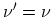, therefore,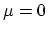(Fig. 2.11-b) and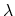can be any of six different phonon polarizations. One can omit the index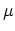and write the phonon frequency as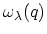and the reduced electron-phonon matrix element for a given band as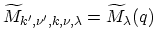, where the weak dependence on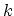is neglected.

For intra-valley processes, most of the phonons have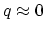and are referred to as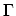-point phonons. Near thepoint a linear dispersion relation for acoustic phonons is assumed,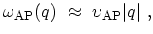(2.16)

where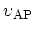is the acoustic phonon velocity. For optical phonons the energy is assumed to be independent of the phonon wave-vector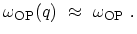(2.17)

Near the-point the reduced electron-phonon matrix elements can be approximated by(2.18)

for acoustic phonons and by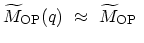(2.19)

for optical phonons . Phonons inducing inter-valley processes have a wave-vector of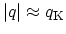, where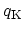is a wave-number corresponding to the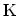-point of the Brillouin zone of graphite. For such phonons one can neglect the q-dependence,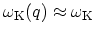and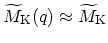.

To calculate the electron-phonon matrix elements one can employ the orthogonal tight-binding , the non-orthogonal tight-binding , and density functional theory  for the band-structure and a force constant model for the lattice dynamics [59,12]. Electron-phonon matrix elements depend on the chirality and the diameter of the CNT [57,56,58]. Figure 2.12 shows the reduced matrix elements for intra-subband intra-valley transitions in semiconducting zigzag and chiral CNTs as a function of the CNT radius .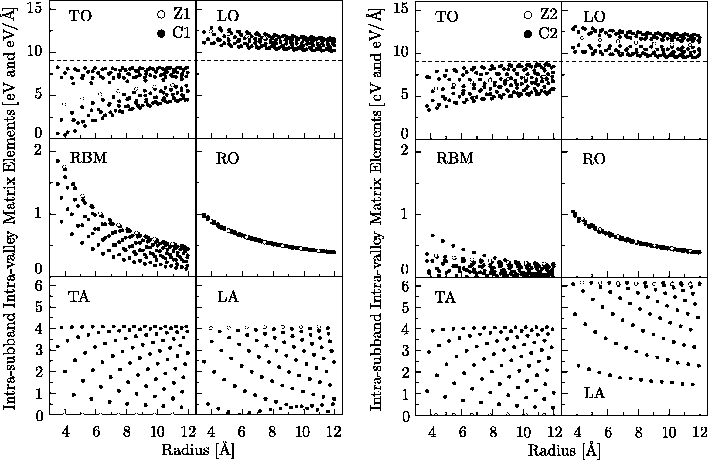M. Pourfath: Numerical Study of Quantum Transport in Carbon Nanotube-Based Transistors